# Using Scientific Calculators Chapter Exam

Exam Instructions:

Choose your answers to the questions and click 'Next' to see the next set of questions. You can skip questions if you would like and come back to them later with the yellow "Go To First Skipped Question" button. When you have completed the practice exam, a green submit button will appear. Click it to see your results. Good luck!

### Page 1

#### Question 1 1. For these questions, I strongly recommend using either the CLEP calculator (found online) or one you use in class. Find x.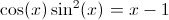#### Question 3 3. For these questions, I strongly recommend using either the CLEP calculator (found online) or one you use in class. Find x.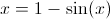#### Question 4 4. For these questions, I strongly recommend using either the CLEP calculator (found online) or one you use in class. At what is the minimum value of f(x) between 0 and 1?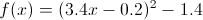#### Question 5 5. For these questions, I strongly recommend using either the CLEP calculator (found online) or one you use in class. Where is the maximum value of f(x) between 0 and pi?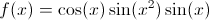### Page 2

#### Question 7 7. The following graph is which of the functions below?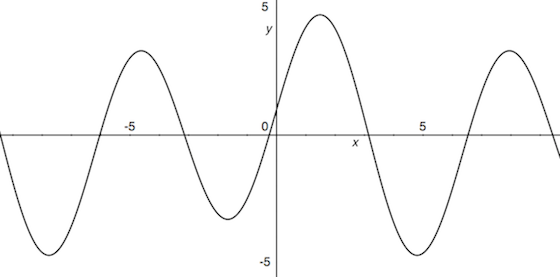#### Question 10 10. For these questions, I strongly recommend using either the CLEP calculator (found online) or one you use in class. Find x.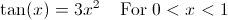### Page 3

#### Question 11 11. For these questions, I strongly recommend using either the CLEP calculator (found online) or one you use in class. Find x.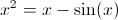### Page 5

#### Question 23 23. For these questions, I strongly recommend using either the CLEP calculator (found online) or one you use in class. What is the value of x where the following two lines intersect?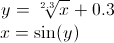#### Question 24 24. For these questions, I strongly recommend using either the CLEP calculator (found online) or one you use in class. What is the root of f(x)? (where f(x) is equal to zero)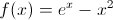### Page 6

#### Question 27 27. For these questions, I strongly recommend using either the CLEP calculator (found online) or one you use in class. Find x.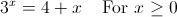#### Using Scientific Calculators Chapter Exam Instructions

Choose your answers to the questions and click 'Next' to see the next set of questions. You can skip questions if you would like and come back to them later with the yellow "Go To First Skipped Question" button. When you have completed the practice exam, a green submit button will appear. Click it to see your results. Good luck!

Support